A variable is a name given to a storage location in which a value is stored in the form of a number or text.

1. Why use Variables?
Variables allow you to change seemingly static values on the fly. Almost all item properties in K-Touch allow you to assign a variable instead of using a fixed value.
1. Where to Place a Variable
• In this example, click a text label in the K-Touch Builder. The figure below shows the properties of that label.
• Each value that can be a variable has a button on the right-hand side. Click this button to assign a variable name to the field.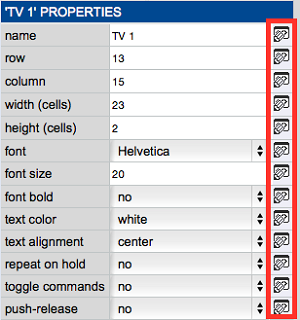1. Assign a Variable
• To assign a variable to a specific property, click the button to the right of that property.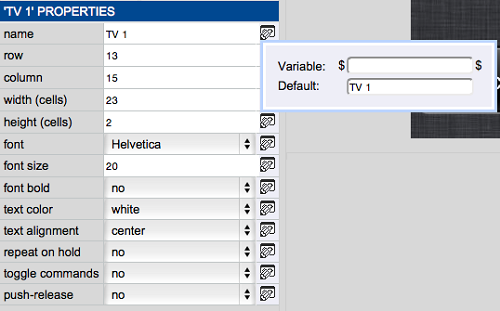• Type a variable name that references this value. In this example, the variable is named ‘label’. Since in K-Touch, all variables are written as \$variable\$, we use \$label\$.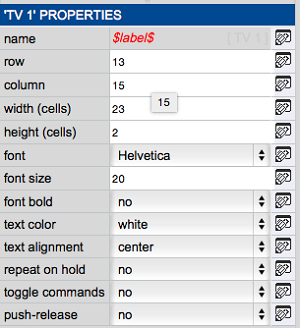1. Set Variable
Now a variable is created for the name of the text label called \$label\$, we can set that variable to another value in our configuration. The value of the variable is set to a default value until it is changed.
• To set a variable, use the set variable action found in the More tab under Actions.
• Drag the set variable action to any element in the GUI.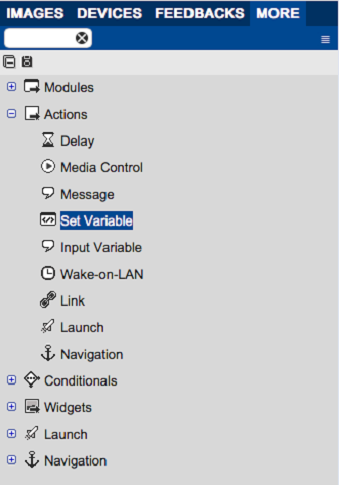1. Set Variable Properties
• After dragging the set variable action to a UI element, set its properties.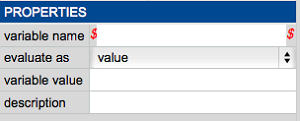Variable Name: The name of the variable to change.
Evaluate As:
Value – Completely change the value of a variable. All text variable changes and some numeric value changes use value evaluations.
Expression – Base the new value of a variable on a mathematical expression. Expressions are for numeric values only.
Variable Value:
If set to value, type the new value of the variable.
If set to expression, type the mathematical equation that calculates the new value (ex. \$var\$ – 1).
Description: An optional free text field that describes the action.